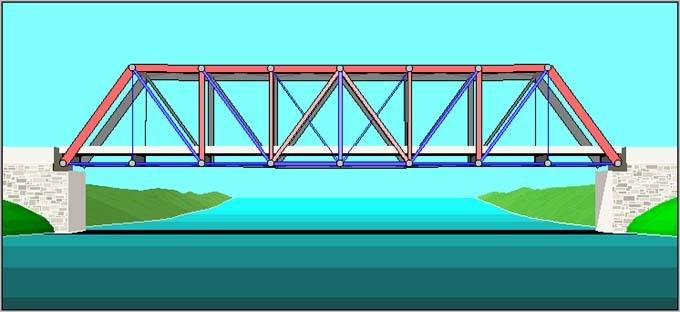# Truss Bridge Tension and Compressions

noahsc

## Homework Statement

Given the weight of a CL-625 design truck (625 kN) on a truss bridge of span 50m, divided into 8 sections (each 6.25 m) with a height of 6.25m making 45deg angles and a width of 6m, what are the tension and compression forces of the members?Mb = PL/4
T=M/H

## The Attempt at a Solution

Using these equations, I got a total tension of 625kN.. I don't know if I'm using the equations wrong or I'm missing something completely, but that seems like a very small number.

## Answers and Replies

Homework Helper
Gold Member
You are not using correct equations....Trusses are subjected primarily to tensile and compressive stresses in each member from member forces under the applied loads, whereas Beams are subject to bending stresses from bending moments under the applied loading. Two different processes. You need to find the force in each member...where is the load placed?

noahsc
yeah im going to restate the title.
this is only part of the problem. what i need to find is the area of the lower and upper chords with the equation T = phi*As*Fy with the Fy=350Mpa

So, what I have been instructed on doing is find the bending moment of the live load M=PL/4 and then find the tension using the moment arm of the height (or vertical truss)